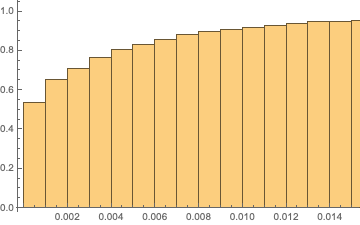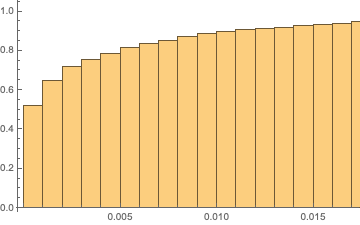# Limited sum for whole sum approximation

Let $$d_n, n\in\{1,2,\cdots,N\}$$ be $$N$$ realizations drawn independent and identically from uniform distribution on $$(0,L)$$ where $$L=\gamma\sqrt{N}$$ with constant $$\gamma$$. Suppose that we need to approximate the sum $$\alpha=\sum_{n=1}^{N}d_n^{-3},$$ with the restricted sum $$\hat{\alpha}=\sum_{n\in\mathcal I}d_n^{-3},$$ where $$\mathcal I\subset N$$. We define the set $$\mathcal I$$ as the largest $$|\mathcal I|$$ element of random variables $$d_1^{-3},d_2^{-3},\cdots,d_N^{-3}$$. Now, the question is to find the order of size of subset $$\mathcal{I}$$ which produce a good approximation ($$\hat{\alpha}\simeq\alpha$$), i.e., $$\mathbb{P}[|\alpha-\hat{\alpha}|\leq\epsilon]\geq 1 -\beta.$$ Is $$|\mathcal I|$$ in order of sub-linear respect to $$N$$?

• If you miss even a single realization you may have an error as large as $1/\epsilon^3$ with probabiity $\epsilon/L$ that vanishes only linearly in $\epsilon$, so this will never provide a good approximation. – Carlo Beenakker Jan 25 at 15:14
• But, this occurs with a very small probability. By choosing $|\mathcal{I}|$ largest value of $d_n^{-3}$, it seems that by properly choosing $|\mathcal{I}|$ we have a good approximation. – Math_Y Jan 25 at 15:21
• But the probability that all of $N$ points be in $(0,\epsilon)$ is $(\epsilon/L)^N$ which is so small. – Math_Y Jan 25 at 15:39
• For example, assume that $N$ points are $L/N,2L/N,\cdots,L$. Then, the question is that is it possible to truncate the series $\sum_{i=1}^{N}i^-3$ to have a good approximation? – Math_Y Jan 25 at 15:43
• It is computationally efficient for me to choose only a small fraction of them. – Math_Y Jan 25 at 15:44

(Edited after noticing an error which completely changes the answer.)

This is not a complete solution; however, it strongly suggests that the answer is positive.

Let $$U$$ be a random variable with uniform distribution on $$[0, 1]$$. The random variable $$U^{-3}$$ has tail $$\mathbb{P}[U^{-3} > x] = x^{-1/3}$$, and hence it is in the domain of attraction of a stable law with index $$\alpha = \tfrac{1}{3}$$.

Observe that $$d_n = L U_n = \gamma \sqrt{N} U_n$$ for an i.i.d. sequence $$U_n$$ with uniform distribution on $$[0, 1]$$. By the invariance principle, the processes $$X^N_t = \frac{1}{N^3} \sum_{n = 1}^{\lfloor N t \rfloor} U_n^{-3}$$ converge (in the appropriate Skorokhod topology) to the increasing $$\tfrac{1}{3}$$-stable Lévy process (i.e. the $$\tfrac{1}{3}$$-stable subordinator) $$X_t$$. Clearly, $$\sum_{n = 1}^{\lfloor N t \rfloor} d_n^{-3} = \frac{N^{3/2} X^N_t}{\gamma^3} \, .$$ Denote $$J = |\mathcal{I}|$$, and let $$\hat\alpha_N$$ be the sum of $$J$$ largest variables among $$d_n^{-3}$$, $$n = 1, 2, \ldots, N$$. Then $$\hat\alpha_N \approx \frac{N^{3/2}}{\gamma} \times (\text{sum of J largest jumps of X_t, t \in [0, 1]}) .$$ (I am not rigorous here. However, I am rather convinced one can turn this into a completely argument.)

The question thus turns into the following problem, with $$\alpha = \tfrac{1}{3}$$ and $$\delta = \tfrac{3}{2}$$:

How many largest jumps of the $$\alpha$$-stable subordinator $$X_t$$, $$t \in [0, 1]$$, one has to add in order to get an approximation which is within $$\pm\gamma N^{-\delta} \times \epsilon$$ from $$X_1$$ with probability $$1 - \beta$$.

This has been studied a lot: it is the question how fast does the Ferguson–Klass–LePage series of a stable subordinator converge. In particular, since the $$n$$-th largest jump of $$X_t$$ is comparable with $$n^{-1/\alpha}$$, the error should be of the order $$J^{1 - 1/\alpha}$$ when $$J$$ largest jumps are taken into account. This suggests that $$J \approx N^{\delta / (1/\alpha - 1)}$$ should do the job. In our case, this gives $$J \approx N^{(3/2) / (3 - 1)} = N^{3/4}$$, and thus it suggests that it is sufficient to take roughly $$|\mathcal{I}| = N^{3/4}$$ largest values of $$d_n^{-3}$$.

I bet one can find a reference for what is written above (and in fact at least some authors do work with Pareto-distributed jumps $$U_n^{-1/\alpha}$$ rather than the ordered jumps of $$X_t$$). I am not an expert in this area, though, and I failed to find a reference in a (very) quick Internet search. The closest one that I encountered is:

Bentkus, V., Juozulynas, A. & Paulauskas, V. Lévy–LePage Series Representation of Stable Vectors: Convergence in Variation. Journal of Theoretical Probability 14, 949–978 (2001)

One may also search in the standard reference for simulation of stable random variables:

Janicki, A., and Weron, A. (1994). Simulation and Chaotic Behaviour of $$\alpha$$-stable Stochastic Processes. Marcel Dekker, New York

If we first choose $$d_n=\frac{L}{nN}$$ and include the $$M$$ smallest $$d_n$$'s in the restricted sum, then the relative error is $$E=\frac{\sum_{n=M+1}^N 1/n^3}{\sum_{n=1}^N 1/n^3}=\frac{\psi ^{(2)}(M+1)}{\psi ^{(2)}(1)}+{\cal O}(N^{-2}).$$ This ratio of polygamma functions amounts to less than a 1% error for $$M=6$$, independent of $$N$$.

For a statistical test I compared $$N=50$$ and $$N=500$$ at a fixed $$M=10$$: shown below are the two histograms of the cumulative distribution of the error $$E$$, obtained from $$10^3$$ realizations of the set of random variables $$d_1,d_2,\ldots d_N$$. As you can see, the two histograms ($$N=50$$ on the left, $$N=500$$ on the right) are nearly the same, with $$E<1\%$$ happening with probability 0.9, so you can keep $$M$$ fixed as you scale up $$N$$.• Thank you so much. I only said $nL/N$ as an example. Maybe, it is better to write the problem in probabilistic way. I mean that $\mathbb{P}(|\alpha-\bar{\alpha}|\leq\beta)$. – Math_Y Jan 25 at 16:22